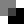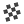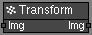# Transform node

Left Toolbar >Compose Nodes >Transform

The Transform node receives an image as an input and moves, rotates and resizes it based on the transform attributes.

When the Transform node is Active, the Transform Manipulator is displayed.

inputImg

outputImg
The image has been rotated and scaled in X and Y.

## Procedure

### To Transform an image:

1. On the left toolbar choose Compose Nodes > Transform.>2. Drag the Transform icon into the Node Graph.3. Connect the output of an image node into the Img input in the Transform node.

4. Adjust the attributes of the Transform node to transform the output image.

5. In the Image Viewport manipulate the Transform node to transform the output image.

## Inputs

Img
The Image to be moved, rotated and scaled.

## Outputs

Img
The moved, rotated and scaled image.

## Attributes PanelTranslate X
Amount of pixels that the image will be translated in X.

Translate Y
Amount of pixels that the image will be translated in Y.

Angle
Degrees that the image will be rotated.

Scale X
Scales the image along the X axis.

Scale Y
Scales the image along the Y axis.

Compose Nodes

Main Page